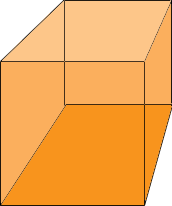SEARCH HOMEMath Central Quandaries & QueriesQuestion from cailtlin, a student: hi.. if the surface area of a cube is 384 cm^2, how could i find the length of one edge? thank youHi Cailtlin,

The surface of a cube is made from 6 congruent squares.What is the area of each square?

What is the formula for the area of a square?

Use the answer to the two previous questions to find the length of an edge.

PennyMath Central is supported by the University of Regina and The Pacific Institute for the Mathematical Sciences.